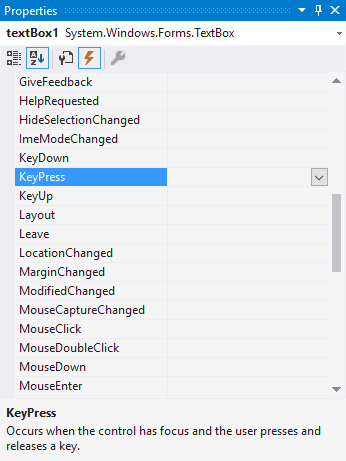Latest articles
• Others
• Others
• Others
• Others
• Others

# How to allow only numbers inside a textbox in Winforms C#

To create an input element on your Windows Form that only accepts numbers, you have 2 options:

## A. Use a NumericUpDown control

If you want to create an input that only accepts number, the first thing that you need to think in is the NumericUpDown control. This controls represents a Windows spin box (also known as an up-down control) that displays exclusively numeric values.

You can simply drag and drop this control from your Toolbox in the All Windows Forms components:Or you can add it dinamically using code:

``````// Create a new numericupdown control
NumericUpDown numbox = new NumericUpDown();
// Some location on the form
numbox.Location = new Point(10, 50);
numbox.Visible = true;
// Append to the form

To retrieve its value you can simply access the Value attribute of the control, for example:

``````// Retrieve numeric up down value
decimal value = numericUpDown1.Value;

MessageBox.Show(value.ToString()); ``````

Note that the value is returned in decimal type, so you can format it into an integer, string or whatever you need. This field by itself doesn't allow non-numeric characters inside.

## B. With a real textbox

In case you aren't able to use a `NumericUpDown` control for some reason, like the usage of a UI framework that only offers a textbox, you can still filter the input by handling properly its `KeyPress` event. The first you need to do is to add a function to the KeyPress event of your input by adding automatically the event with Visual Studio selecting your textbox and in the Properties toolbox (right bottom corner of VS), then selecting the events tab and double clicking the KeyPress option:This will automatically add the KeyPress function on your class that will be empty, then you need to modify it with the following code to prevent the input from non-numeric characters:

``````private void textBox1_KeyPress(object sender, KeyPressEventArgs e)
{
// Verify that the pressed key isn't CTRL or any non-numeric digit
if (!char.IsControl(e.KeyChar) && !char.IsDigit(e.KeyChar) && (e.KeyChar != '.'))
{
e.Handled = true;
}

// If you want, you can allow decimal (float) numbers
if ((e.KeyChar == '.') && ((sender as TextBox).Text.IndexOf('.') > -1))
{
e.Handled = true;
}
}``````

To retrieve its value, you would need only to convert the string to a number by using the desired type:

``````string textboxValue = textBox1.Text;

// Retrieve as decimal
decimal valueDec = decimal.Parse(textboxValue);

// Retrieve as integer
int valueInt = Int32.Parse(textboxValue);``````

Happy coding !